로그인 처리 중...

Trial ends in

Chapter 2

## Summarizing and Visualizing Data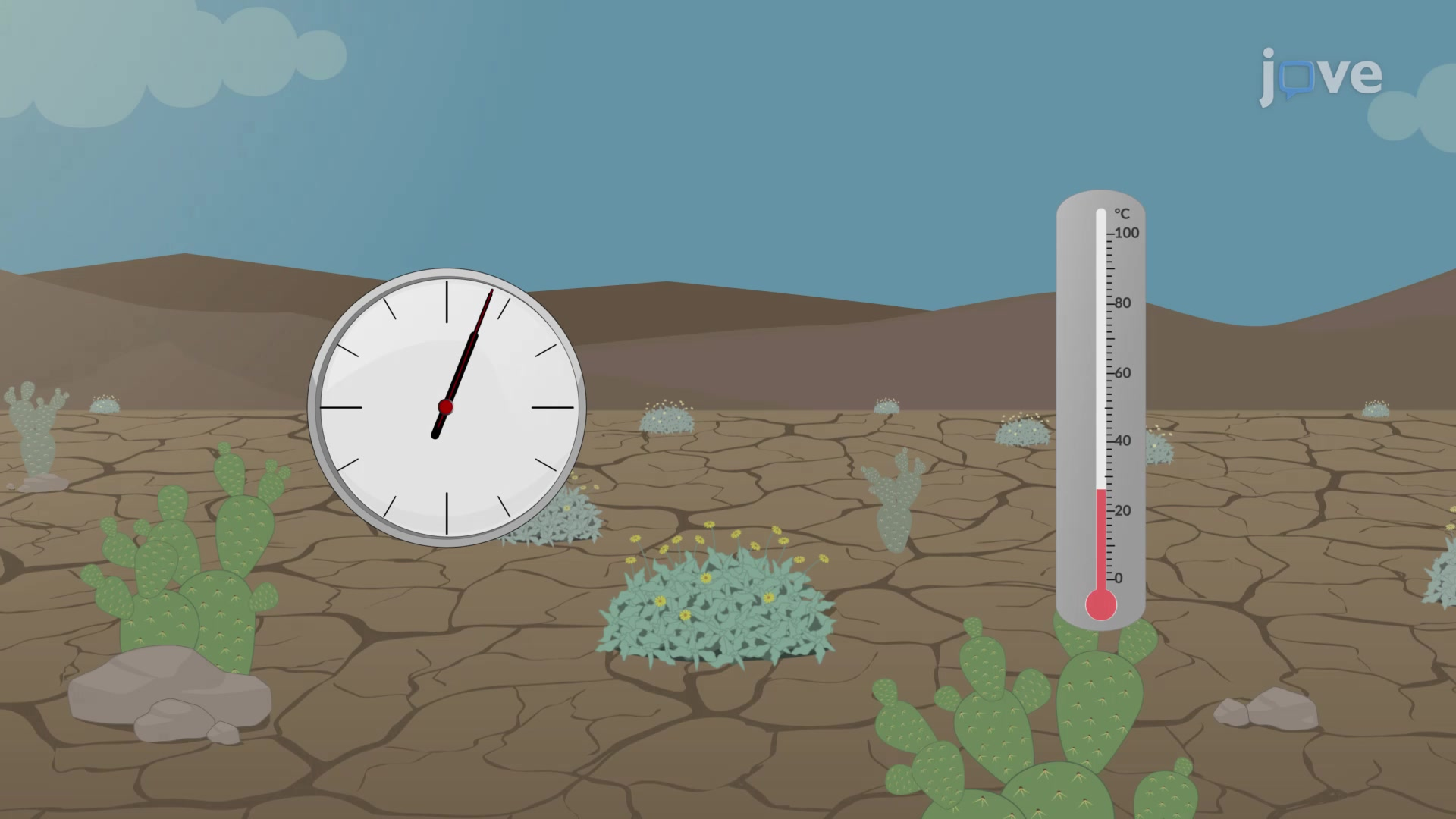Data are individual items of information obtained from a population or sample. Data may be classified as qualitative (categorical), quantitative…A frequency is the number of times a value of the data occurs. The sum of all the frequency values represents the total number of students included…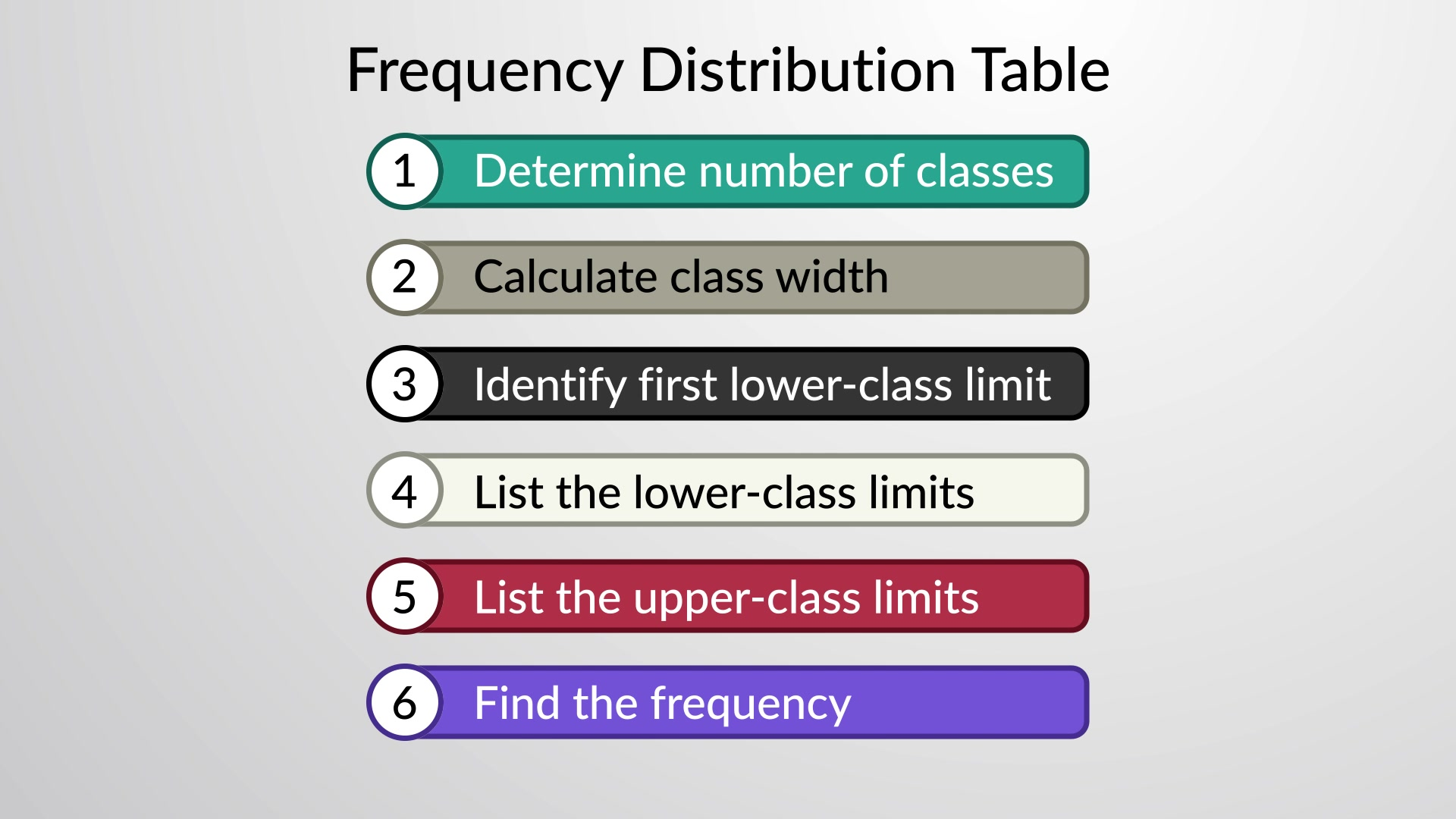A frequency distribution table can be constructed using the steps given below. First, make a table with two columns—one with the title of the…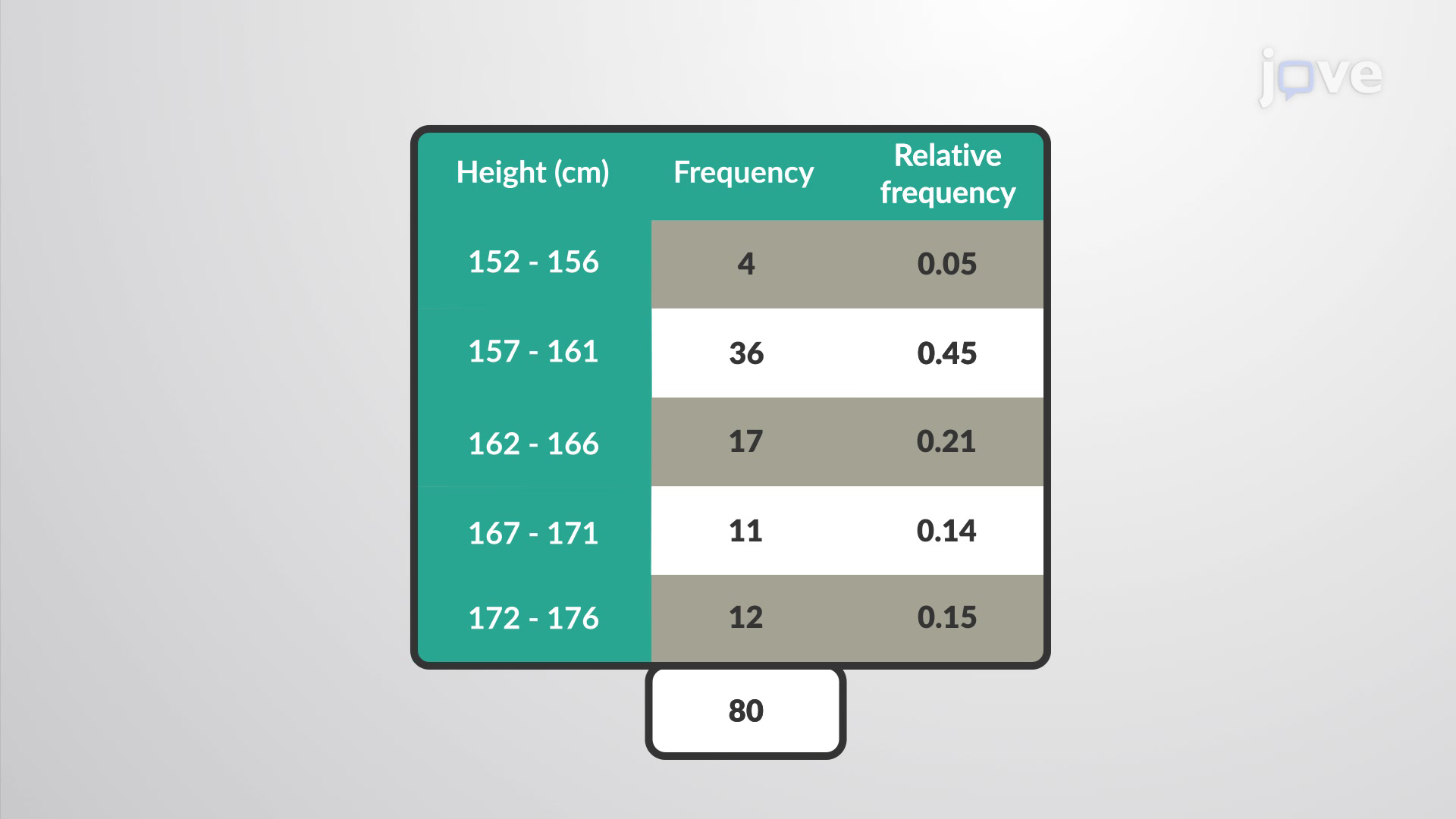A relative frequency distribution is the proportion or fraction of times a value occurs in a data set. To find the relative frequencies, one can…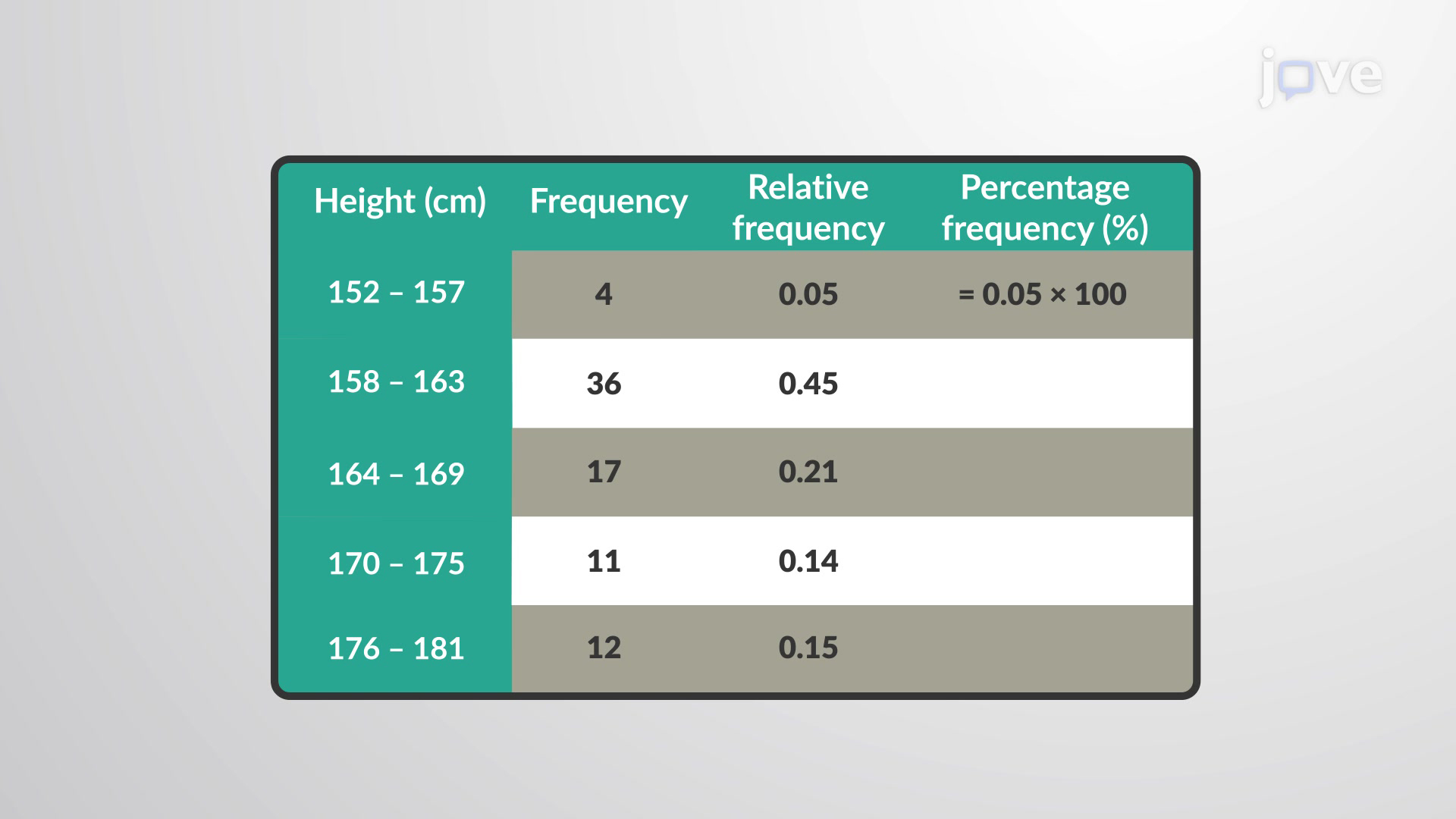A percentage frequency distribution, in general, is a display of data that indicates the percentage of observations for each data point or grouping…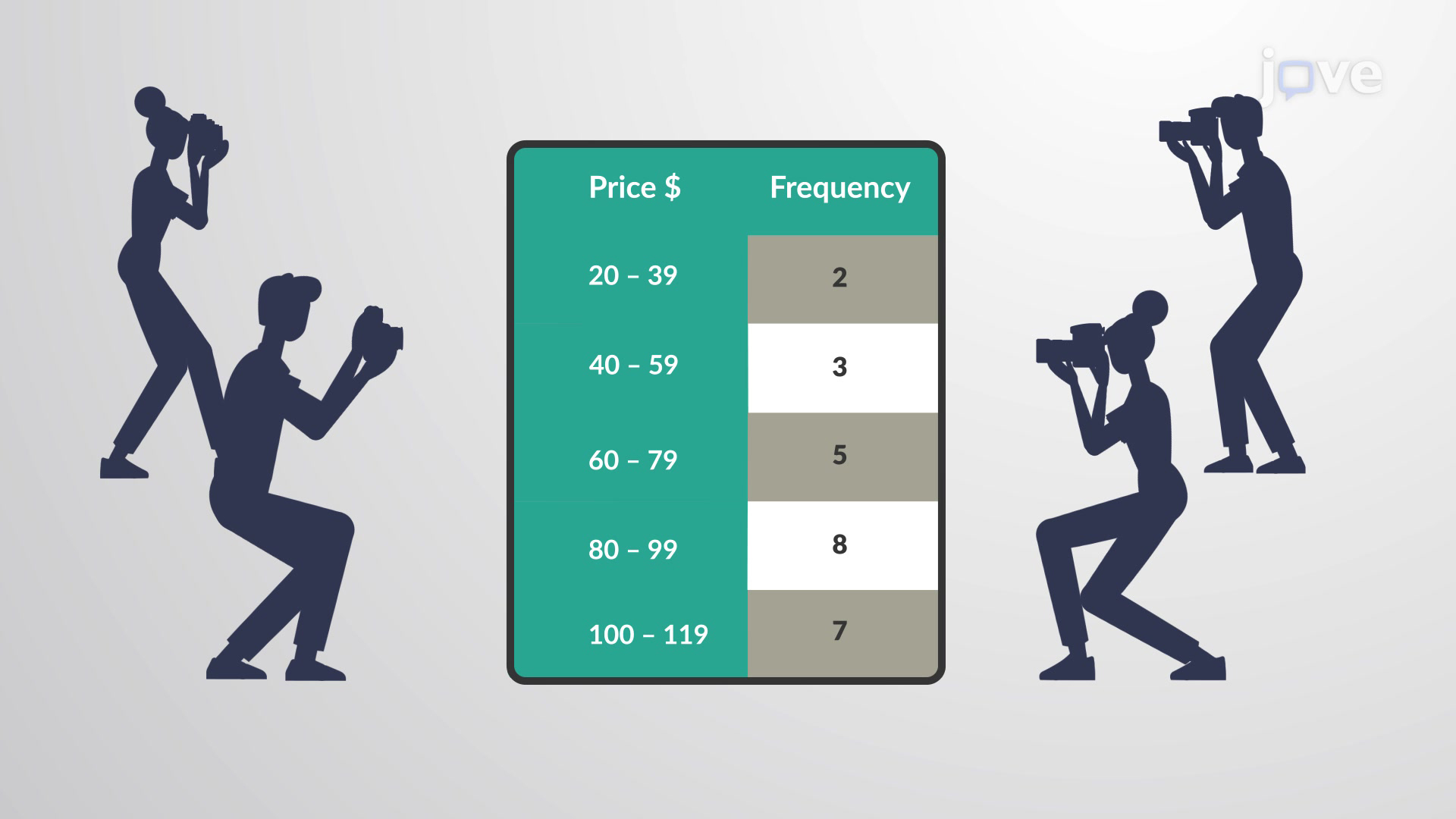A cumulative frequency distribution is another type of frequency distribution. Instead of reporting how many data values fall in some classes, it…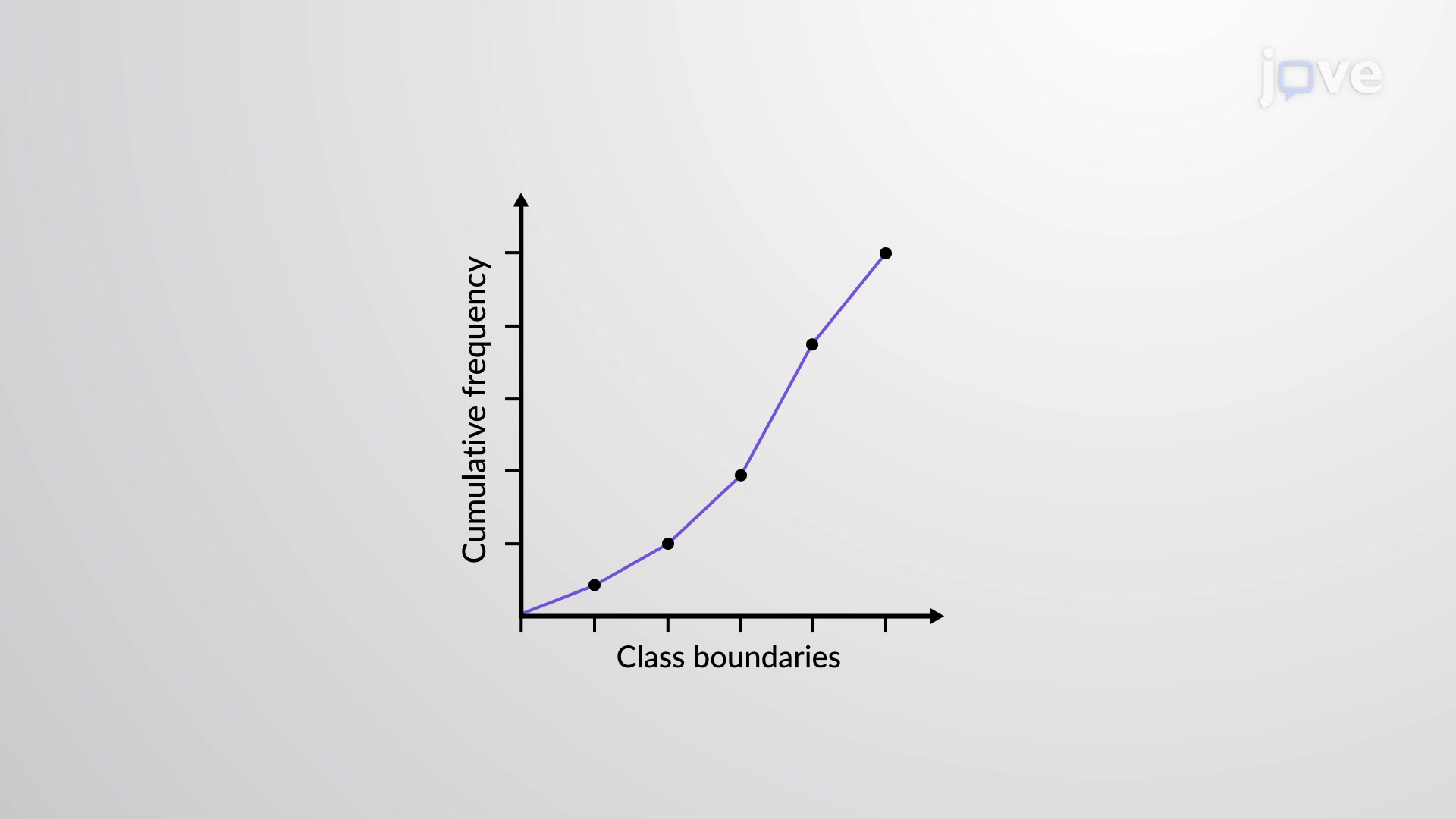An ogive graph is sometimes called a cumulative frequency polygon. It is one type of frequency polygon that shows cumulative frequency. In other…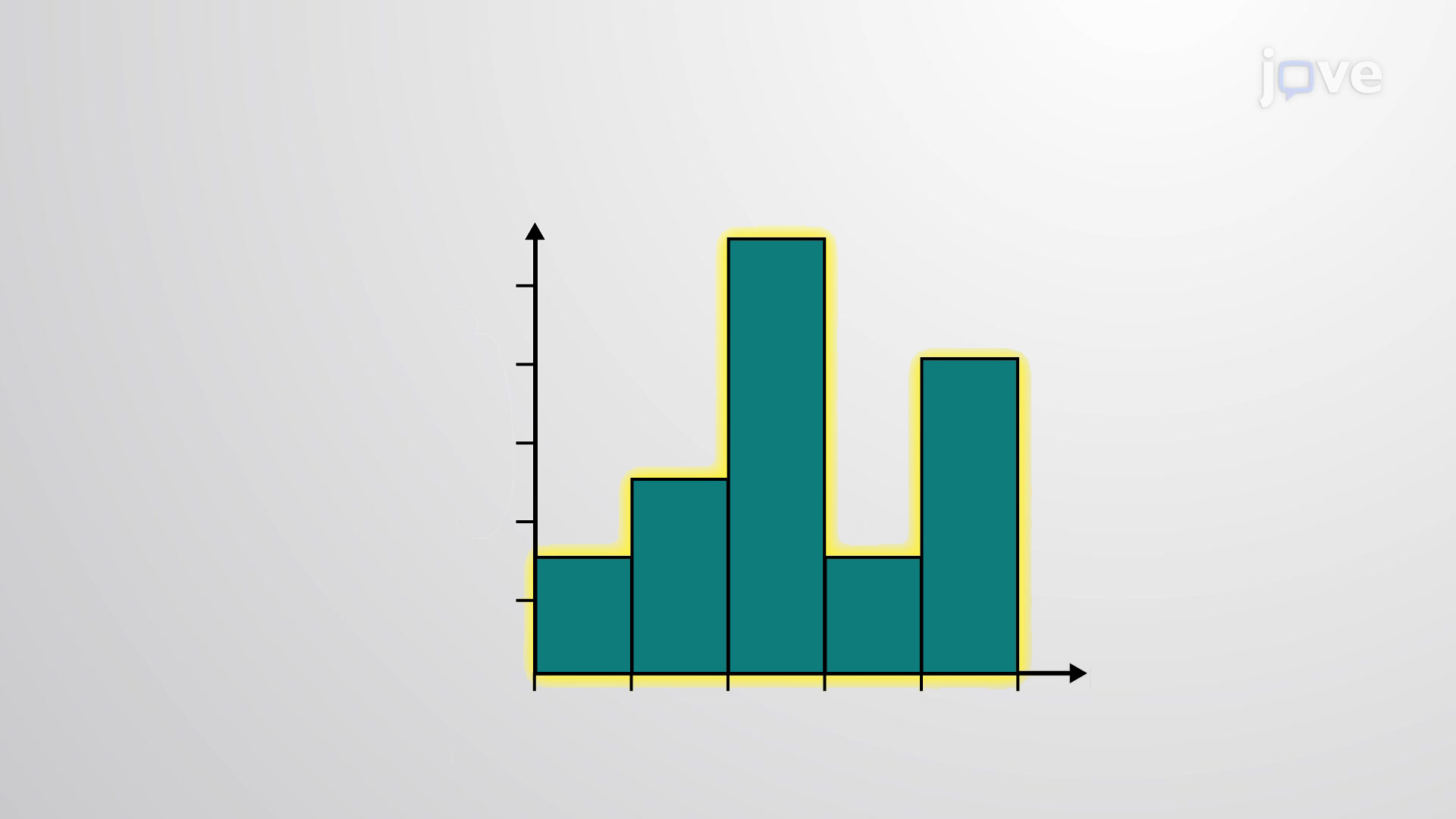The histogram is a graphical representation in the x-y form of data distribution in a data set. The horizontal x-axis is labeled with what the data…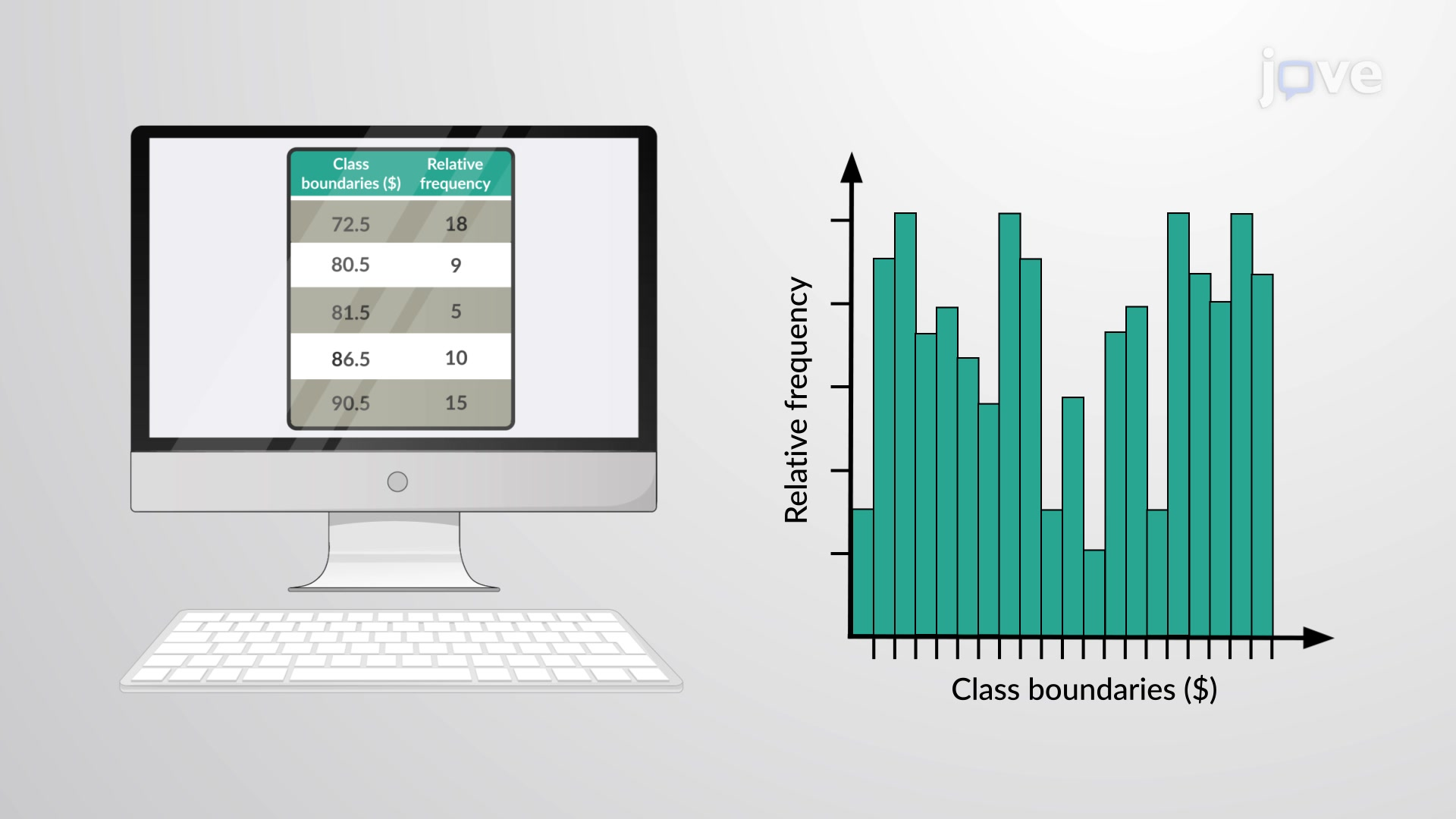The relative frequency depicts the proportion of data points that have each value. The frequency tells the number of data points that have each…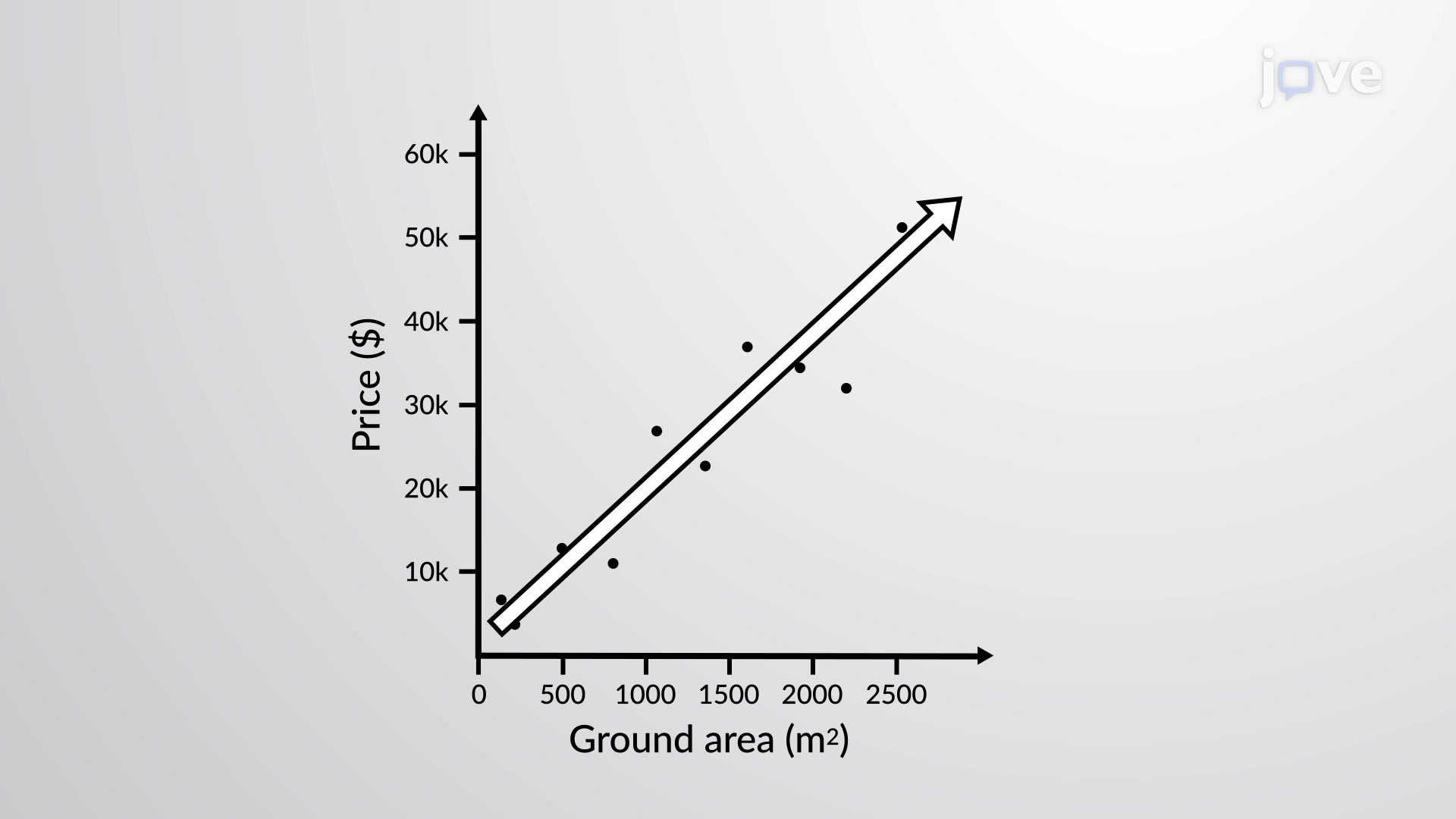The most common and easiest way to display the relationship between two variables, x and y, is a scatter plot. A scatter plot shows the direction of…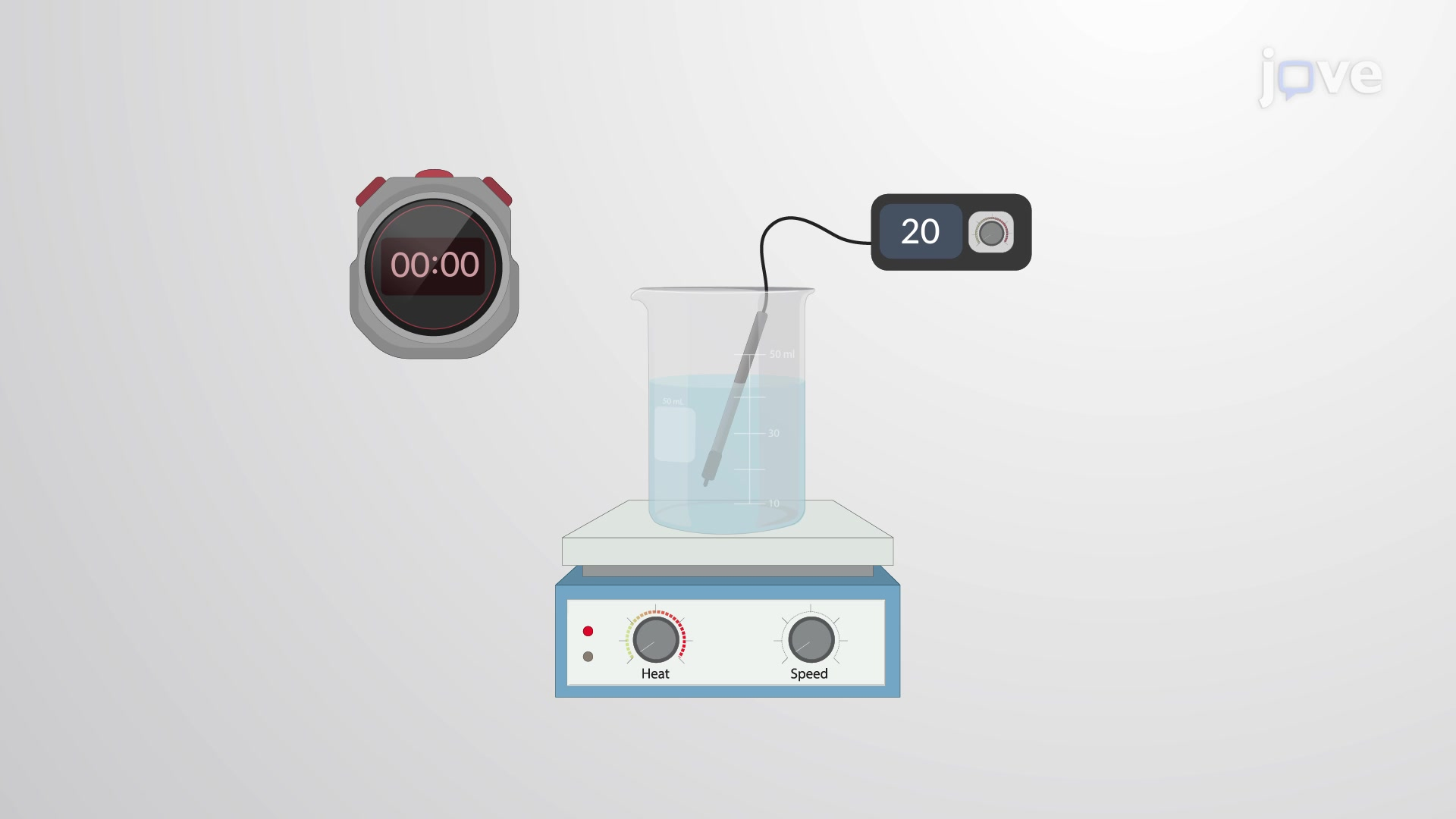A time-series graph is a line graph with repeated measurements taken at successive intervals of time. It is also called a time series chart. To…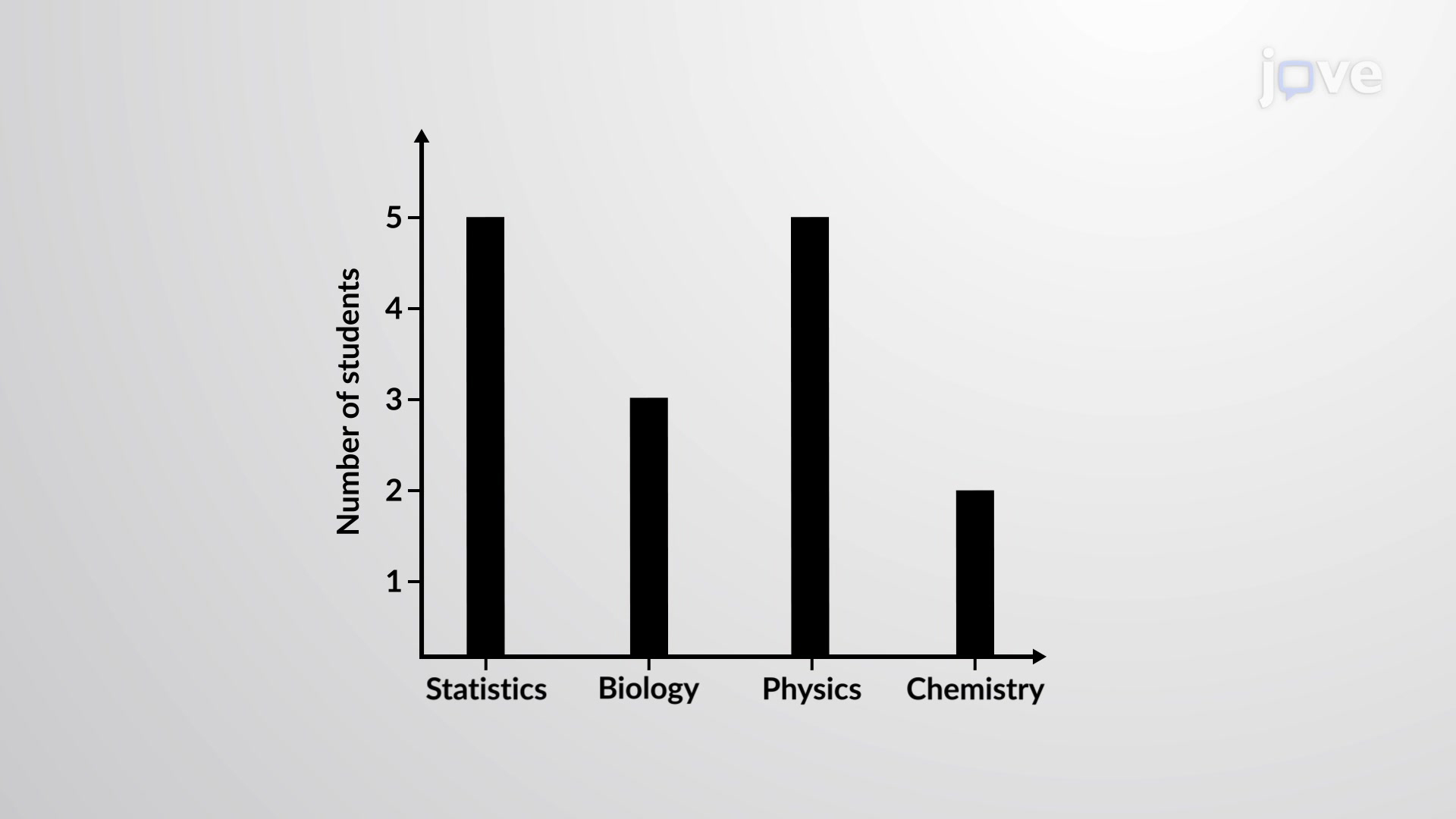A bar graph is also called a bar chart and consists of bars that are separated from each other. It either uses horizontal or vertical bars to show…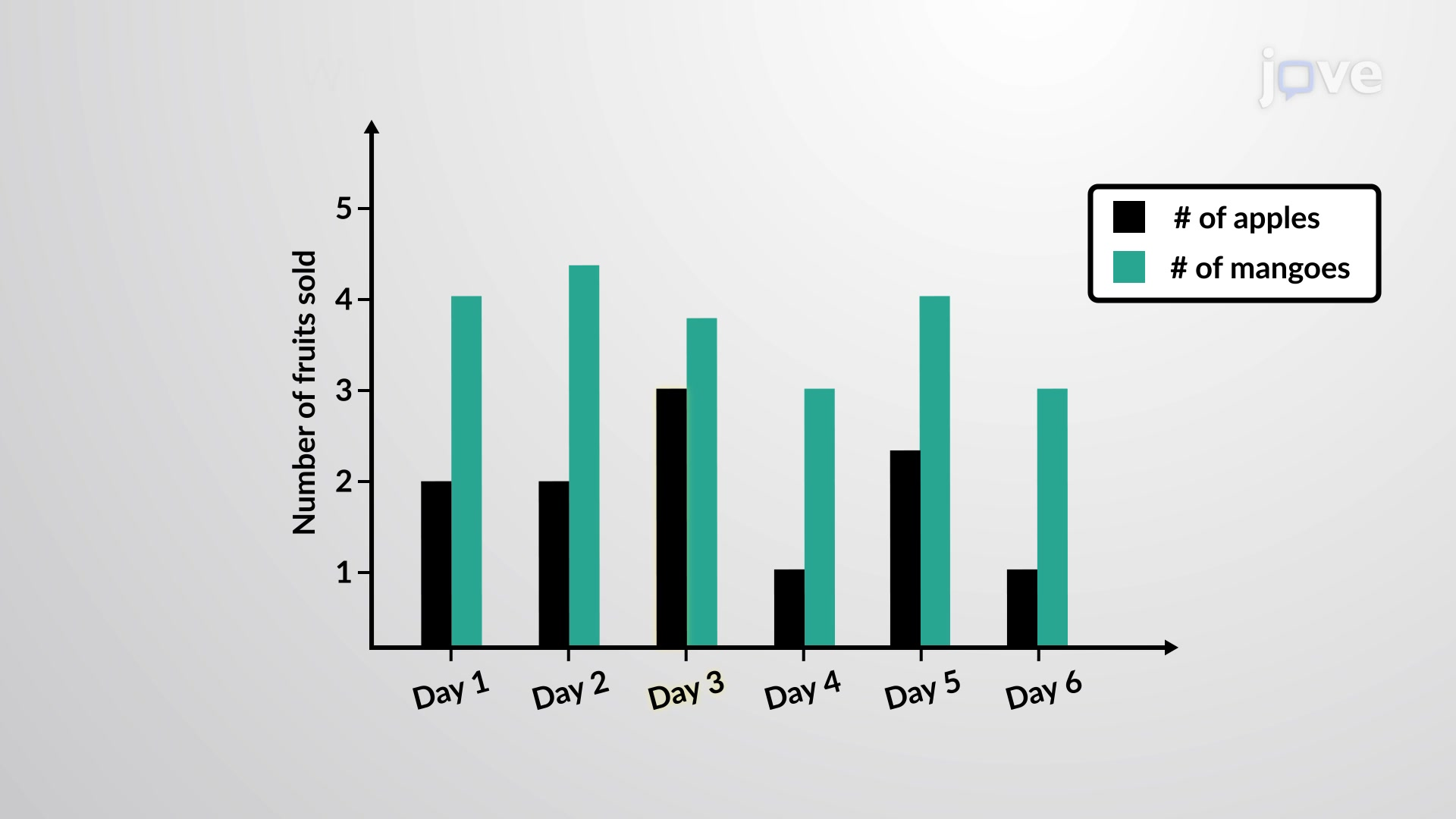As the name suggests, a multiple bar graph is the same as a bar graph but has multiple bars to depict relationships between different data values.…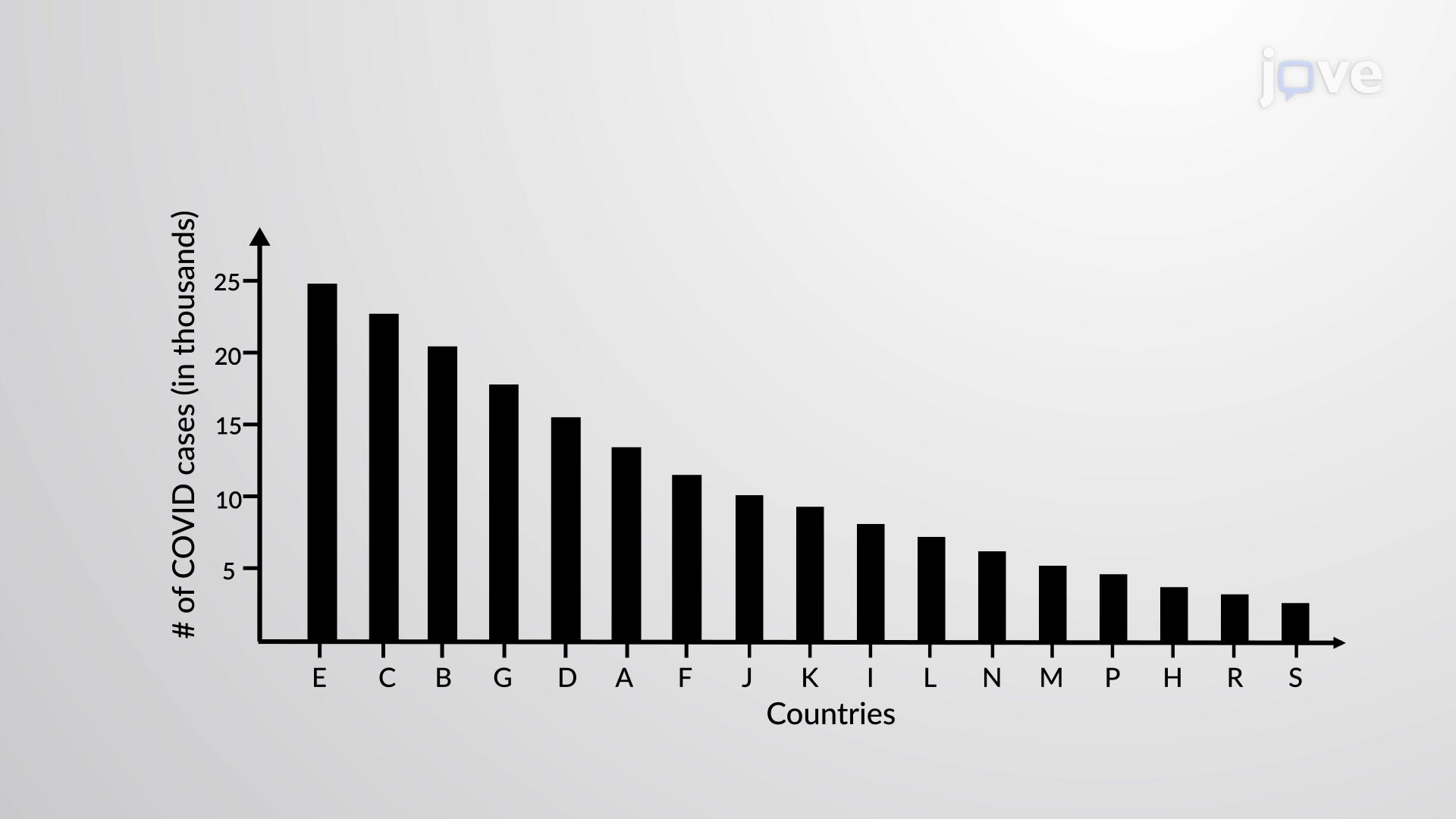A Pareto chart is a bar graph or a combination of both line and bar graphs. The bar lengths represent the individual values or the frequency, while…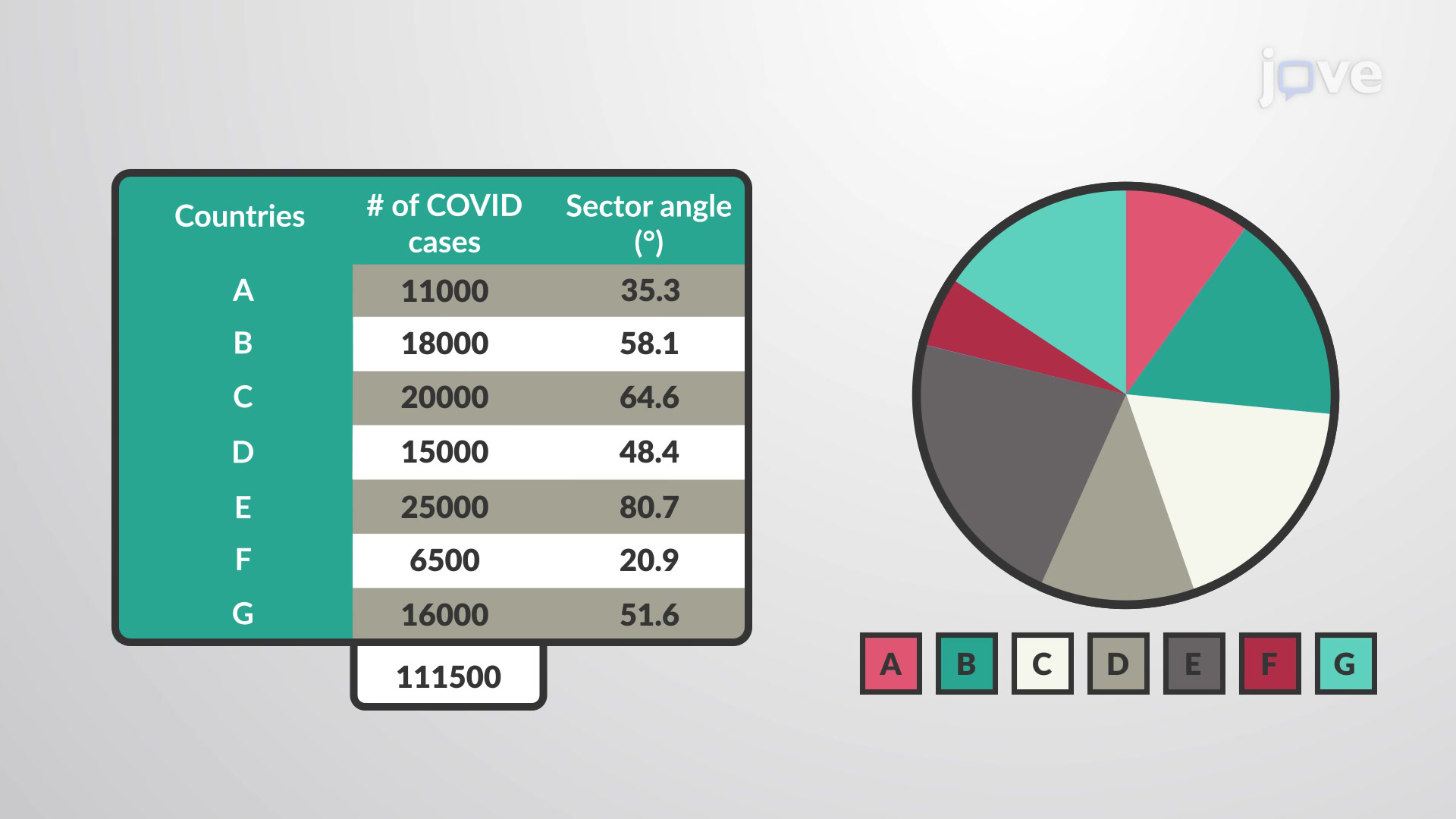A pie chart (or a pie graph) is a circular graphical chart or a pictorial representation of categorical data. It is divided into slices of pie each…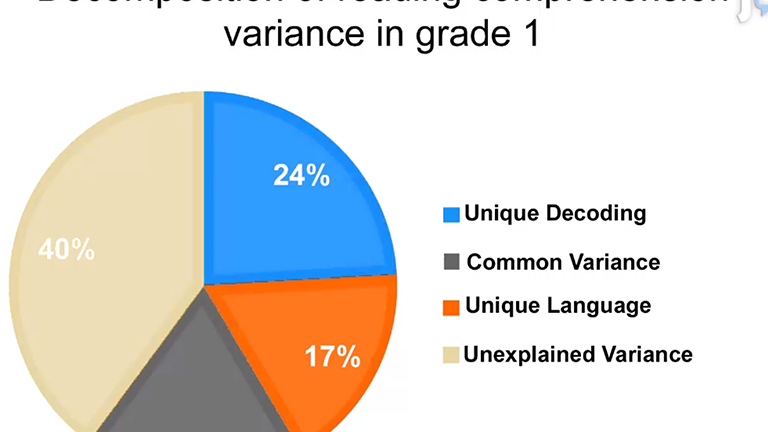The Simple View of Reading is a popular model of reading that claims that reading is the product of decoding and language, with each component…Due to discrepancies between self-reported and observed pro-environmental behavior, researchers suggest the use of more direct measures of behavior.…From a single molecule at a silver nanoaggregate junction, blinking surface-enhanced Raman scattering (SERS) is observed. Here, a protocol is…

### Get cutting-edge science videos from JoVE sent straight to your inbox every month.X